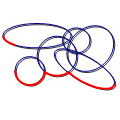CGAL 4.14 - 2D Envelopes
2D Envelopes ReferenceRon Wein
This package consits of functions that computes the lower (or upper) envelope of a set of arbitrary curves in 2D. The output is represented as an envelope diagram, namely a subdivision of the $$x$$-axis into intervals, such that the identity of the curves that induce the envelope on each interval is unique.
Introduced in: CGAL 3.3
Depends on: 2D Arrangements
BibTeX: cgal:w-e2-19a

This package consists of functions that compute the lower (or upper) envelope of a set of arbitrary curves in 2D. The output is represented as an envelope diagram, namely a subdivision of the $$x$$-axis into intervals, such that the identity of the curves that induce the envelope over each interval is unique.

## Functions

• CGAL::lower_envelope_2
• CGAL::upper_envelope_2
• CGAL::lower_envelope_x_monotone_2
• CGAL::upper_envelope_x_monotone_2

## Concepts

• EnvelopeDiagram_1
• EnvelopeDiagramVertex
• EnvelopeDiagramEdge

## Classes

• CGAL::Envelope_diagram_1<Traits>

Concepts

## Classes

class  CGAL::Envelope_diagram_1< Traits >
This class is the default envelope-diagram class used by envelope functions to represent the minimization or the maximization diagram of a set of curves. More...

## Functions

template<class InputIterator , class EnvelopeDiagram >
void CGAL::lower_envelope_2 (InputIterator begin, InputIterator end, EnvelopeDiagram &diag)
Computes the lower envelope of a set of curves in $$\mathbb{R}^2$$, as given by the range [begin, end). More...

template<class InputIterator , class EnvelopeDiagram >
void CGAL::lower_envelope_x_monotone_2 (InputIterator begin, InputIterator end, EnvelopeDiagram &diag)
Computes the lower envelope of a set of $$x$$-monotone curves in $$\mathbb{R}^2$$, as given by the range [begin, end). More...

template<class InputIterator , class EnvelopeDiagram >
void CGAL::upper_envelope_2 (InputIterator begin, InputIterator end, EnvelopeDiagram &diag)
Computes the upper envelope of a set of curves in $$\mathbb{R}^2$$, as given by the range [begin, end). More...

template<class InputIterator , class EnvelopeDiagram >
void CGAL::upper_envelope_x_monotone_2 (InputIterator begin, InputIterator end, EnvelopeDiagram &diag)
Computes the upper envelope of a set of $$x$$-monotone curves in $$\mathbb{R}^2$$, as given by the range [begin, end). More...

## ◆ lower_envelope_2()

template<class InputIterator , class EnvelopeDiagram >
 void CGAL::lower_envelope_2 ( InputIterator begin, InputIterator end, EnvelopeDiagram & diag )

#include <CGAL/envelope_2.h>

Computes the lower envelope of a set of curves in $$\mathbb{R}^2$$, as given by the range [begin, end).

The lower envelope is represented using the output minimization diagram diag.

Template Parameters
 InputIterator must be an input iterator with value type EnvelopeDiagram::Traits_2::Curve_2. EnvelopeDiagram must be a model of the concept EnvelopeDiagram_1.
Examples:
Envelope_2/envelope_circles.cpp.

## ◆ lower_envelope_x_monotone_2()

template<class InputIterator , class EnvelopeDiagram >
 void CGAL::lower_envelope_x_monotone_2 ( InputIterator begin, InputIterator end, EnvelopeDiagram & diag )

#include <CGAL/envelope_2.h>

Computes the lower envelope of a set of $$x$$-monotone curves in $$\mathbb{R}^2$$, as given by the range [begin, end).

The lower envelope is represented using the output minimization diagram diag.

Template Parameters
 InputIterator must be an input iterator with value type EnvelopeDiagram::X_monotone_curve_2. EnvelopeDiagram must be a model of the concept EnvelopeDiagram_1.
Examples:
Envelope_2/convex_hull_2.cpp, and Envelope_2/envelope_segments.cpp.

## ◆ upper_envelope_2()

template<class InputIterator , class EnvelopeDiagram >
 void CGAL::upper_envelope_2 ( InputIterator begin, InputIterator end, EnvelopeDiagram & diag )

#include <CGAL/envelope_2.h>

Computes the upper envelope of a set of curves in $$\mathbb{R}^2$$, as given by the range [begin, end).

The upper envelope is represented using the output maximization diagram diag.

Template Parameters
 InputIterator must be an input iterator with value type EnvelopeDiagram::Traits_2::Curve_2. EnvelopeDiagram must be a model of the concept EnvelopeDiagram_1.
Examples:
Envelope_2/envelope_circles.cpp.

## ◆ upper_envelope_x_monotone_2()

template<class InputIterator , class EnvelopeDiagram >
 void CGAL::upper_envelope_x_monotone_2 ( InputIterator begin, InputIterator end, EnvelopeDiagram & diag )

#include <CGAL/envelope_2.h>

Computes the upper envelope of a set of $$x$$-monotone curves in $$\mathbb{R}^2$$, as given by the range [begin, end).

The upper envelope is represented using the output maximization diagram diag.

Template Parameters
 InputIterator must be an input iterator with value type EnvelopeDiagram::X_monotone_curve_2. EnvelopeDiagram must be a model of the concept EnvelopeDiagram_1.
Examples:
Envelope_2/convex_hull_2.cpp.